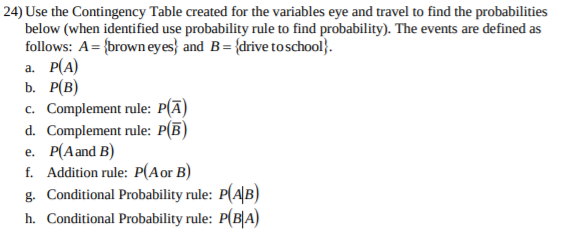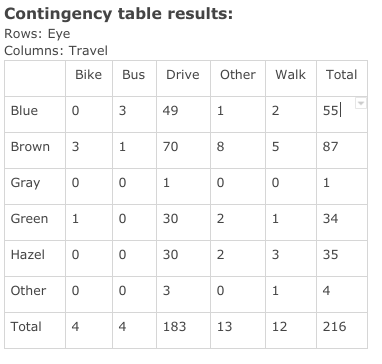# 24) Use the Contingency Table created for the variables eye and travel to find the probabilitiesbelow (when identified use probability rule to find probability). The events are defined asfollows: A={brown eyes} and B{drive to school}а. Р(А)b. Р(В)c. Complement rule: P(Ad. Complement rule: P(Be. P(Aand B)f. Addition rule: P(Aor B)g. Conditional Probability rule: P(AB)h. Conditional Probability rule: P(B|A) Contingency table results:Rows: EyeColumns: TravelBikeOtherWalkTotalBusDrive55Blue34912387Brown17085Gray001103034Green12.1Hazel30352Other01Total441831312216Lс

Question
25 viewshelp_outlineImage Transcriptionclose24) Use the Contingency Table created for the variables eye and travel to find the probabilities below (when identified use probability rule to find probability). The events are defined as follows: A={brown eyes} and B{drive to school} а. Р(А) b. Р(В) c. Complement rule: P(A d. Complement rule: P(B e. P(Aand B) f. Addition rule: P(Aor B) g. Conditional Probability rule: P(AB) h. Conditional Probability rule: P(B|A) fullscreenhelp_outlineImage TranscriptioncloseContingency table results: Rows: Eye Columns: Travel Bike Other Walk Total Bus Drive 55 Blue 3 49 1 2 3 87 Brown 1 70 8 5 Gray 0 0 1 1 0 30 34 Green 1 2. 1 Hazel 30 35 2 Other 0 1 Total 4 4 183 13 12 216 Lс fullscreen
check_circle

Step 1

Given data is:

 Travel Bike Bus Drive Other Walk Total Eye Blue 0 3 49 1 2 55 Brown 3 1 70 8 5 87 Gray 0 0 1 0 0 1 Green 1 0 30 2 1 34 Hazel 0 0 30 2 3 35 Other 0 0 3 0 1 4 Total 4 4 183 13 12 216

Let A is brown eyes and B is drive to school.

a.

See the above given table,

Total number of brown eyes or in the other world says favorable outcome = 87

Total outcome = 216

Now, the probability P(A) is calculated as follows:

Step 2

b.

See the above given table,

Total number of drive to school or in the other world says favorable outcome = 183

Total outcome = 216

Now, the probability P(B) is calculated as follows:

Step 3

### Want to see the full answer?

See Solution

#### Want to see this answer and more?

Solutions are written by subject experts who are available 24/7. Questions are typically answered within 1 hour.*

See Solution
*Response times may vary by subject and question.
Tagged in

### Statistics